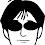# Java Program on Swapping Two Numbers Without Using Third Variable

Swapping two numbers without using third or temporary variable is one of the most commonly asked question not just on Java but also on C and C++ tests and interviews. Swapping two numbers or strings is a very easy question till the condition of using only two variable is not applied, lots of freshers failed to solve this swap without using the third variable. Mostly we come out with a solution with just arithmetic operator but if the test level is little up and asks about other approaches of swapping two variables without using third or temp variable, than you have to look over this little program.
Since you are looking for a swap two numbers program in java without using any temp variable, let me tell that this post is a complete resource to learn different approaches on swapping two numbers numbers without using third variable.

Description:

We just have to swap(interchange) the 2 numbers, stored in 2 different variables. Don't forget we are not using the third variable.
example:
INPUT>   x=12 & y=53
OUTPUT> x=53 & y=12

As I told you earlier, you may be asked to swap two numbers with different approaches without using any temporary or third variable. This post contains four methods to do so!

Method 1: Swapping Using Arithmetic Operator
In 90% of cases this method strikes first to a fresher while swapping without using third variable.
```import java.io.*;
class swap{
public static void main(String args[]) throws IOException{
System.out.print("Enter 1st no: ");
System.out.print("Enter 2nd no: ");
System.out.println("\nNumbers Before Swapping:");
System.out.println("x = "+x);
System.out.println("y = "+y);
//Swapping Method 1
x=x+y;
y=x-y;
x=x-y;
System.out.println("\nNumbers After Swapping:");
System.out.println("x = "+x);
System.out.println("y = "+y);
}
}
```

Method 2: Swapping Using Bitwise Operators
Second approach two swap to numbers without using third variable is using XOR bitwise operators.
```import java.io.*;
class swap{
public static void main(String args[]) throws IOException{
System.out.print("Enter 1st no: ");
System.out.print("Enter 2nd no: ");
System.out.println("\nNumbers Before Swapping:");
System.out.println("x = "+x);
System.out.println("y = "+y);
//Swapping Method 2
x = x^y;
y = x^y;
x = x^y;
System.out.println("\nNumbers After Swapping:");
System.out.println("x = "+x);
System.out.println("y = "+y);
}
}
```

Method 3: Swapping Using Multiplication & Division
Like  Method 1 where we swapped two numbers x and y without using third variable by the simple logic of addition and subtraction, In this method we do the same swapping using multiplication and division logic keeping in mind that we don't have to use third variable.
```import java.io.*;
class swap{
public static void main(String args[]) throws IOException{
System.out.print("Enter 1st no: ");
System.out.print("Enter 2nd no: ");
System.out.println("\nNumbers Before Swapping:");
System.out.println("x = "+x);
System.out.println("y = "+y);
//Swapping Method 3
x = x*y;
y = x/y;
x = x/y;
System.out.println("\nNumbers After Swapping:");
System.out.println("x = "+x);
System.out.println("y = "+y);
}
}
```

Method 4: Swapping Using 'One Line Logic!'
Now here comes my favorite method to swap two numbers... Its the best method for swapping because only one line logic is required for this. i.e,  x = y-x+(y=x);
```import java.io.*;
class swap{
public static void main(String args[]) throws IOException{
System.out.print("Enter 1st no: ");
System.out.print("Enter 2nd no: ");
System.out.println("\nNumbers Before Swapping:");
System.out.println("x = "+x);
System.out.println("y = "+y);
//Swapping Method 4
x = y-x+(y=x);
System.out.println("\nNumbers After Swapping:");
System.out.println("x = "+x);
System.out.println("y = "+y);
}
}
```

OUTPUT:So that's it guys now you know four ways to swap two variables without using third variable in Java. Its good to know multiple ways to swap two variables without using temporary or third variable.
Now its your time to show your response, If you liked this post and feels you learned something great than hit the like/+ button below, share this awesome articles with your friends and let they know these three approaches which we can apply while swapping two numbers without using third variable.

If I forgotten something or you know any other approach, do let us know. Having problem in swapping number in Java or any other program comment you question below!import java.io.*;
public class InterChange
{
public static void main()throws IOException
{
int x, y, z;
System.out.println("Enter the Value of x");
System.out.println("Enter the Value of y");
System.out.println("Before InterChanging");
System.out.println("x value="+x);
System.out.println("y value="+y);
z=y;
y=x;
x=z;
System.out.println("After InterChanging");
System.out.println("x value="+x);
System.out.println("y value="+y);
}
}Hello Maneesh...
Thanks for sharing your program for swapping using 3 variables :)I knew only the mutliplication and divison one . Thanks for telling more.Glad to know you liked our post! Keep visiting....This comment has been removed by the author.one line logic is outstanding sir ..thank u